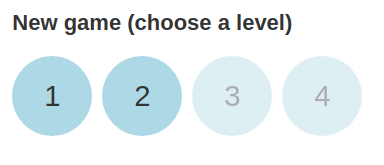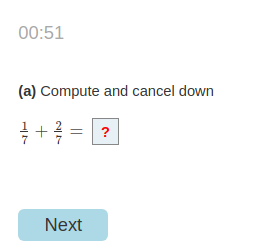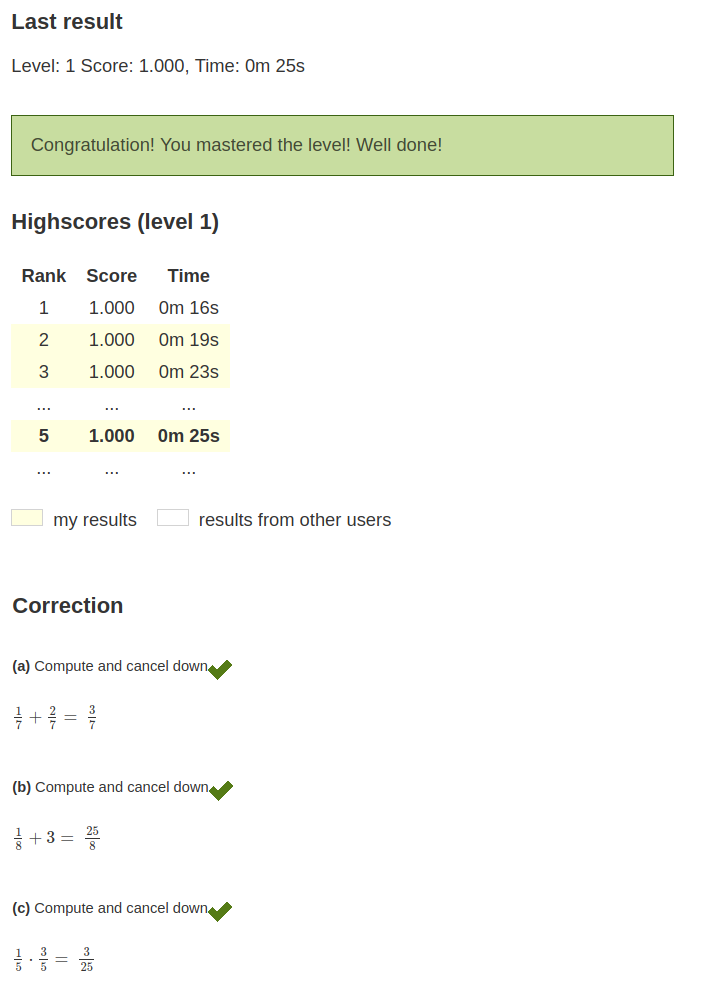Overview

Working with MUMIE as author

Working with MUMIE as teacher

Go back to the old wiki

We revise and update this wiki. We apologize for the inconvenience this may cause you.

# How to create a game

## Serious gaming

Games are one way to include playful components in your teaching material. It's easy to achieve that within our generic framework.

• Use the already known syntax to define questions: How to create a problem document. Use different types of questions (multiple choice, input questions, ...), define variables, include images and audio, add explanations and so on.
• Configure the game with a few easy commands. Set a maximum time for a level or for the questions. Set the the threshold score to unlock the next level and decide if a user can repeat a level he or she already completed successfully
• Define as many game levels as you like. Choose from the questions you've defined.

## User workflow

As a user you first choose a level to start a game. Starting a game for the first time, only level 1 is unlocked. A timer starts running, counting down to zero or just counting the seconds a user needs to finish if no limit is set. The user answers the questions one by one. The game ends either when a time-out is reached or the user has answered the last question of a level. All questions are immediately and automatically corrected. The users see the correction, the score and needed time as well as a (anonymous) highscore list for the selected level with the top results from all users.

choose a level play the game see your result, the correction and the highscore list## Code example

The example game above is created by the following code. Only selected code fragment are shown. For the full example with live preview see: complete example

1234567891011121314151617181920212223242526272829303132333435363738394041\begin{problem} \duration[q]{60}\levelthreshold{0.8}\levelrepeatable % first level\begin{level}  \question{1}  \question{2}  \randomquestionpool{5}{6}  % [...]further question references[...]\end{level} % second level\begin{level}  % [...]question references[...]\end{level} % [...]further levels[...] % question 1\begin{question}    \text{Compute and cancel down}    \begin{variables}        \randrat{a}{1}{1}{5}{9}        \randint{b}{2}{4}        \function[calculate]{c}{a*b}        \function[calculate]{d}{a+c}    \end{variables}    \type{input.number}    \field{rational}    \begin{answer}        \text{$\var{a} + \var{c} =$ }        \solution{d}    \end{answer}\end{question} % [...]further questions[...] \end{problem} 

## Game-specific environments and commands

name type usage description
level environment \begin{level}...\end{level} Inside a 'level' environment you can define which questions are used for this level and you can set level specific options (e.g. max duration).
question command \question{n} where n is the index of the question Define a question you want to use as part of a level. The command is short for \randomquestionpool{n}{n} (see Randomquestionpool).
duration command \duration[q]{t} where t is the time in seconds. Define the max time (in seconds) a user has for all questions of a level combined. Use the optional parameter 'q' to declare that the t is the max time for each question instead of for all questions together. If the duration is not set there will be no time limit at all for the user to answer the questions.
levelthreshold command \levelthreshold{p} where p is a value between 0 and 1 Define which score is necessary to master a level and unlock the next one.
levelrepeatable command \levelrepeatable Define if the user can repeat a level or not.

Use the generic problem syntax to define variables, tasks, questions and so on: How to create a problem document

## How to use a game within a course

Add the 'gaming' problem to a worksheet. Set the worksheet type to 'game' and declare the worksheet as a wrapper. You can use only one 'gaming' problem per worksheet.